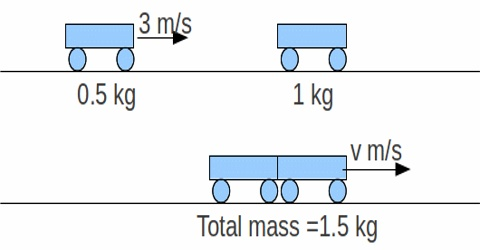# What is Momentum?

It can be said that the motion produced in a body due to the combined effect of mass and velocity is the momentum of that body. Keeping mass constant, if velocity is increased then momentum of the body also increases. If same body moves with higher velocity, then its momentum also becomes higher. As many times the velocity of a body increases same increase of force compared to previous one is needed to stop that body. If a car moves with double velocity, then double amount of force compared to previous one will be needed to stop that car. A bullet is very small but its speed is very large and so momentum becomes large and this impulse of the bullet is very strong.

The property of a body that is produced in it due to the combined effect of mass and velocity of the body is called momentum. It is measured by the product of mass and velocity of the body. Inertia of Motion will proportional to momentum. It is a vector quantity.

Unit: S.I. unit of momentum is, kg ms-1

Dimension: [P] = [MLT-1]